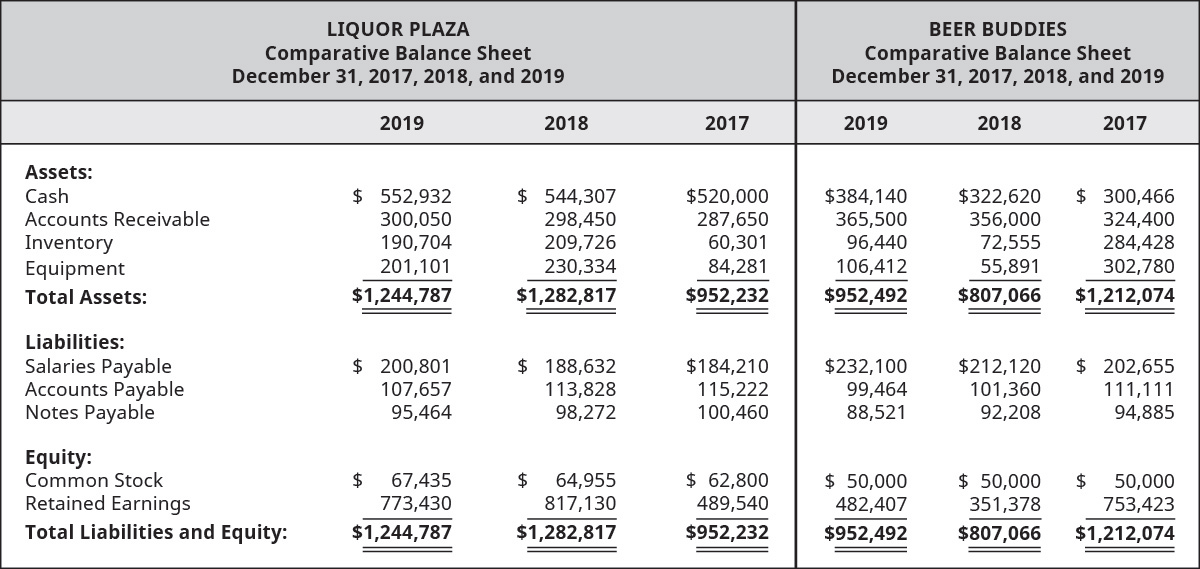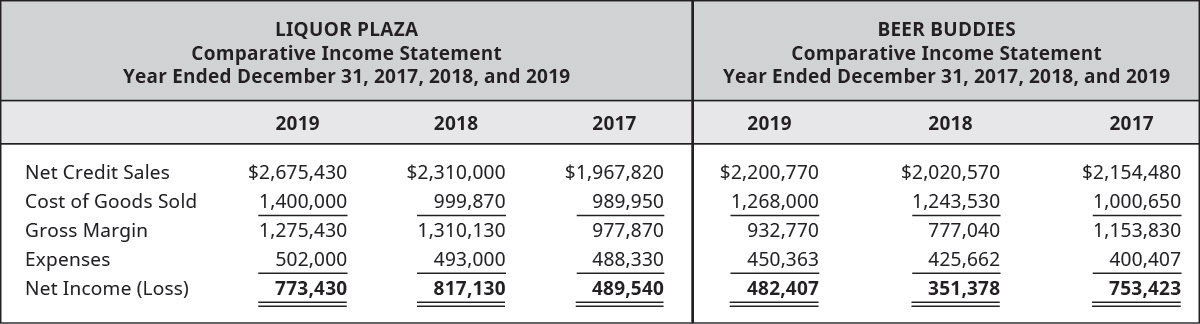# Review the select information for Liquor Plaza and Beer Buddies (industry competitors) and complete the following. A. Compute the accounts receivable turnover ratios for each company for 2018 and 2019. B. Compute the number of day’s sales in receivables ratios for each company for 2018 and 2019. C. Determine which company is the better investment and why. Round answers to two decimal places.FindFindarrow_forward

### Principles of Accounting Volume 1

19th Edition
OpenStax
Publisher: OpenStax College
ISBN: 9781947172685

#### Solutions

Chapter
SectionFindFindarrow_forward

### Principles of Accounting Volume 1

19th Edition
OpenStax
Publisher: OpenStax College
ISBN: 9781947172685
Chapter 9, Problem 7PB
Textbook Problem
1 views

## Review the select information for Liquor Plaza and Beer Buddies (industry competitors) and complete the following.A. Compute the accounts receivable turnover ratios for each company for 2018 and 2019.B. Compute the number of day’s sales in receivables ratios for each company for 2018 and 2019.C. Determine which company is the better investment and why. Round answers to two decimal places.To determine

(a)

Introduction:

The accounts receivables turnover ratio shows the company’s performance with respect to the company’s debt collection and company’s net credit sales. The number of days’ in receivable ratios shows the estimated days to convert accounts receivables into cash.

To calculate:

Account receivable turnover ratio of the companies for the year 2018 & 2019.

### Explanation of Solution

L. Plaza:

Formula to calculate accounts receivable turnover ratio in 2018:

AccountsReceivablesTurnoverRatio=(NetCreditSalesAverageAccountsReceivables)

Substitute, $2,310,000 in net credit sales and$293,050 in average accounts receivables.

AccountsReceivablesTurnoverRatio=($2,310,000$293,0501)=7.88

Therefore, account receivable turnover ratio in 2018 is 7.88.

Formula to calculate accounts receivable turnover ratio in 2019:

AccountsReceivablesTurnoverRatio=(NetCreditSalesAverageAccountsReceivables)

Substitute, $1,400,000 in net credit sales and$299,250 in average accounts receivables.

AccountsReceivablesTurnoverRatio=($1,400,000$299,2502)=4.67

Therefore, account receivable turnover ratio in 2019 is 4.67.

B Buddies:

Formula to calculate accounts receivable turnover ratio in 2018:

AccountsReceivablesTurnoverRatio=(NetCreditSalesAverageAccountsReceivables)

Substitute, $2,020,570 in net credit sales and$340,200 in average accounts receivables.

AccountsReceivablesTurnoverRatio=($2,020,570$340,2003)=5.93

Therefore, account receivable turnover ratio in 2018 is 5

To determine

(b)

To calculate:

Number of days’ sales in receivable ratio of the companies for the year 2018 & 2019.

To determine

(c)

The company which is better among two stated.

### Still sussing out bartleby?

Check out a sample textbook solution.

See a sample solution

#### The Solution to Your Study Problems

Bartleby provides explanations to thousands of textbook problems written by our experts, many with advanced degrees!

Get Started